# Variable Expressions

Variable Expressions

 1 Introduction to Variable Expressions 2 Definition of Variable Constant, Term and Coefficient 3 Variable Expression (Algebraic Expression) 4 Types of Variable Expressions 5 Thinking out of the Box! 6 Activity on Evaluating the Variable Expressions 7 Solved Examples on Variable Expressions 8 Challenging Questions on Variable Expressions 9 Practice Questions on Variable Expressions 10 Important Topics 11 Maths Olympiad Sample Papers 12 Frequently Asked Questions (FAQs)

We at Cuemath believe that Math is a life skill. Our Math Experts focus on the “Why” behind the “What.” Students can explore from a huge range of interactive worksheets, visuals, simulations, practice tests, and more to understand a concept in depth.

Book a FREE trial class today! and experience Cuemath's LIVE Online Class with your child.

## Introduction to Variable Expressions

James and Natalie were playing with match sticks and they thought of forming patterns of numbers using the matchsticks.

James took 4 match sticks and formed the number $$4$$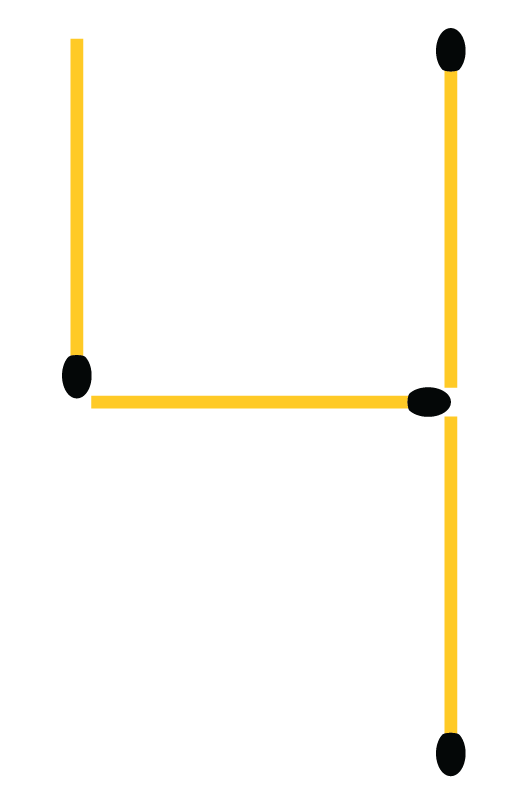Natalie added 3 more match sticks to form a pattern with two $$4$$s.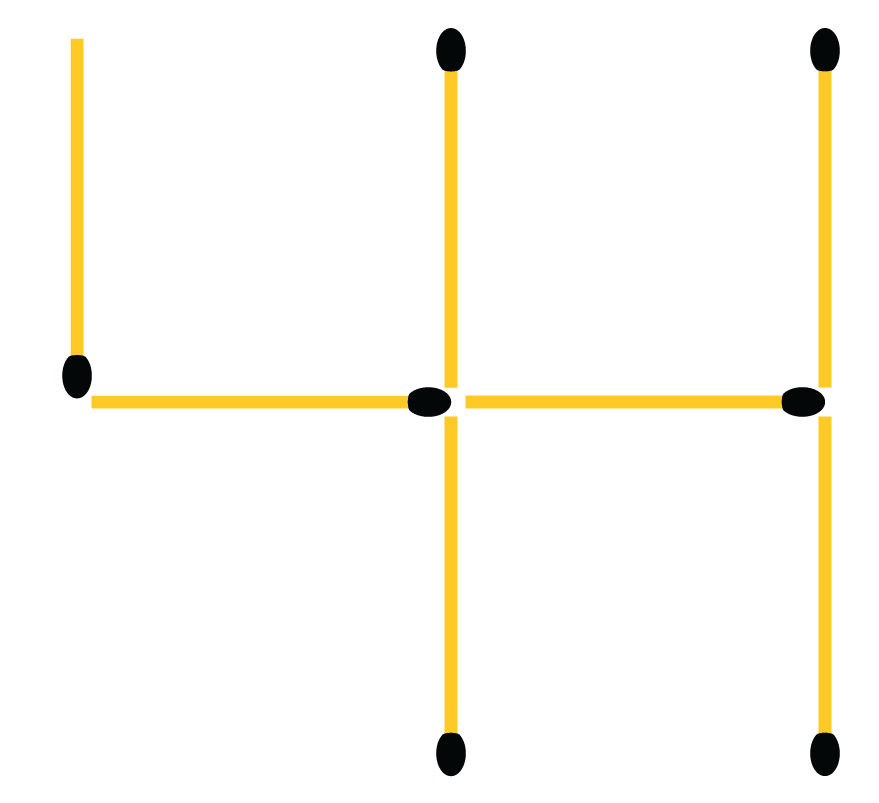Then James again added 3 more match sticks to form a pattern with three $$4$$s.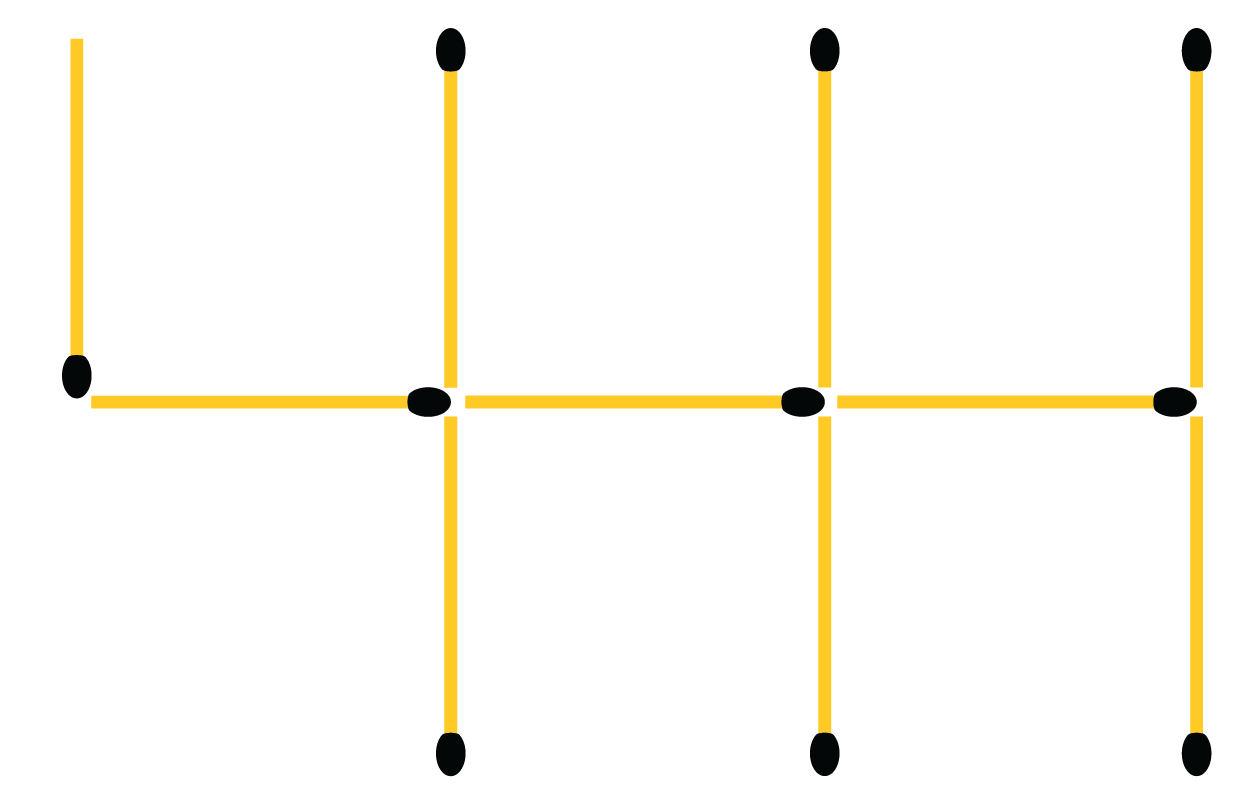Suddenly, Natalie got a doubt that how many match sticks are required to make a pattern of ten $$4$$s?

They understood from the existing pattern that they need $$4+ 9 (3)$$ sticks to get it done as they want a pattern with ten $$4$$s.

From this, they concluded that they need $$4+(n-1)3$$ sticks, in general, to make a pattern with $$n$$ number of $$4$$s.

Here, $$4+(n-1)3$$ is called an algebraic expression.

## Definition of Variable, Constant, Term and Coefficient

• A symbol that doesn't have a fixed value is called a variable in Math. It can take any value.

In the above example, $$n$$ is a variable and here it can take the values $$1,2,3,...$$

Some examples of variables in Math are $$a,b, x, y, z, m,$$ etc.

• A symbol that has a fixed numerical value is called a constant.

All numbers are constants.

Some examples of constants are $$3, 6, \dfrac{-1}{2}, \sqrt{5}$$ etc.

• A term is a variable alone (or) a constant alone (or) it can be a combination of variables and constants by the operation of multiplication or division.

Some examples of terms are $$3x^2, \dfrac{-2}{3}y, \sqrt{5m},$$ etc.

Here, the numbers that are multiplying the variables are $$3, \dfrac{-2}{3}$$ and $$5$$ which are called coefficients.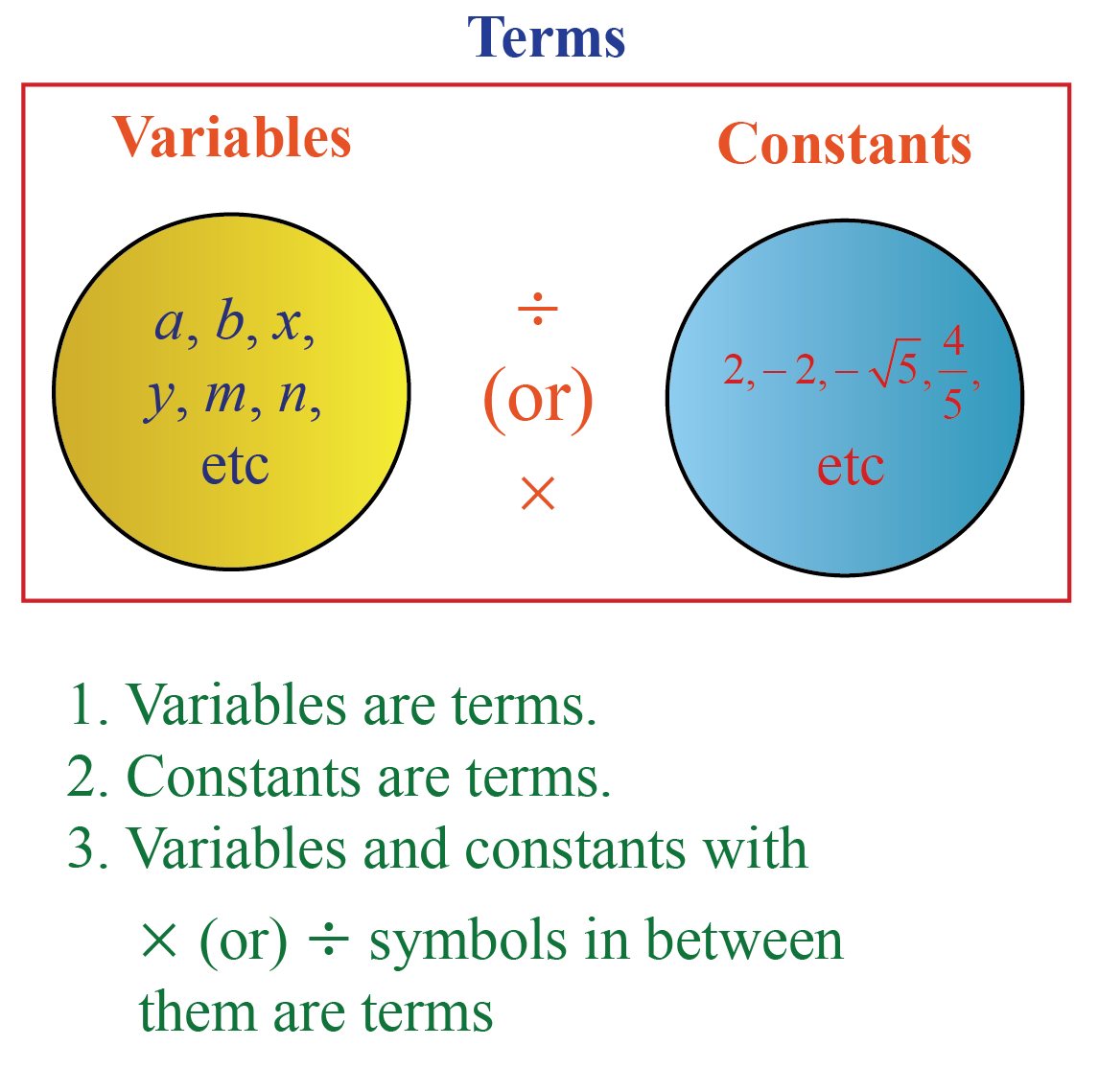CLUEless in Math? Check out how CUEMATH Teachers will explain Variable Expressions to your kid using interactive simulations & worksheets so they never have to memorise anything in Math again!

Explore Cuemath Live, Interactive & Personalised Online Classes to make your kid a Math Expert. Book a FREE trial class today!

## Variable Expression (Algebraic Expression)

A variable expression (or) an algebraic expression is a combination of terms by the operations such as addition, subtraction, multiplication, division, etc.

### Example of a Variable Expression

An example of a variable expression (or) an algebraic expression is $$5x + 7$$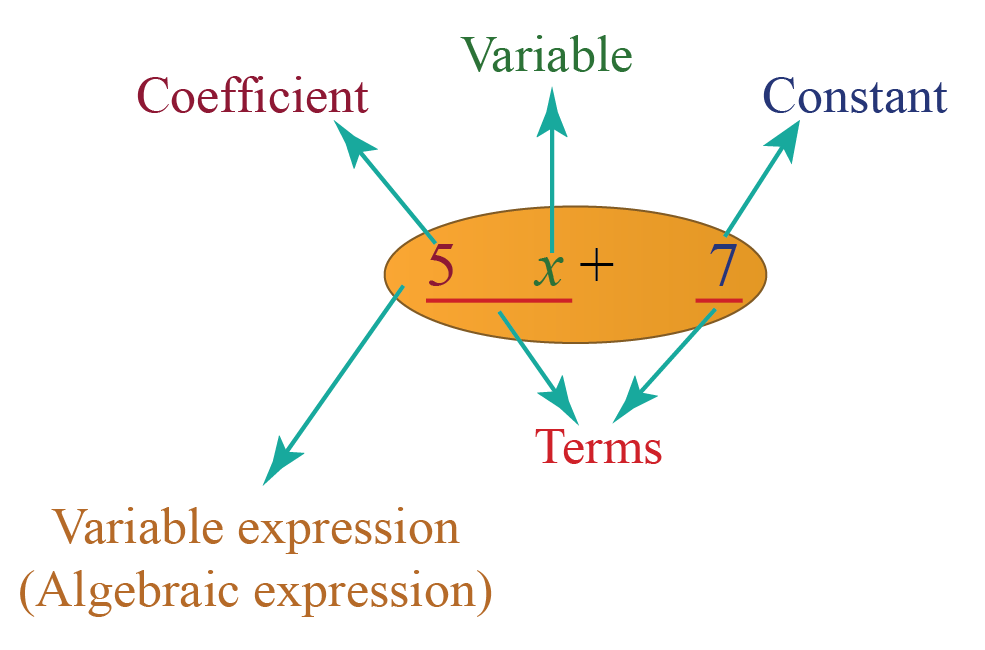### Evaluating a Variable Expression

To evaluate a variable expression at a given value, we just substitute that value in the expression and simplify it.

Examples:

Evaluate $$5x^2+2x+7$$ at $$x= -2$$

Solution:

Substitute $$x=-2$$ in $$5x^2+2x+7$$,

\begin{align}5(-2)^2+2(-2)+7 &= 5(4)-4+7 \\&= 20-4+7\\&= 23\end{align}

So the answer is $$23$$

## Types of Variable Expressions

There are $$5$$ types of variable expressions (or) algebraic expressions.

Types Meaning Examples

Monomial

An expression with only one term where the exponents of all the variables are non-negative integers

$$3xy$$

Binomial

An expression with two monomials

$$\dfrac{3}{4}x - 2y^2$$

Trinomial

An expression with three monomials

$$3x-2y+ z$$

Polynomial

An expression with one or more monomials

$$\dfrac{-2}{3}x^3\!\!+\!7x^2\!+\!3x\!+\!5$$

Multinomial

An expression with one or more terms (the exponents of variables can be either positive or negative)

$$4x^{-1} +2y+3z$$Think Tank
1. Is every polynomial a multinomial?
2. Is every multinomial a polynomial?

## Activity on Evaluating the Variable Expressions

Here is an activity on Variable Expressions.

From this, you can select one of the given variable expressions and give the value(s) for its variable(s).

Then you can evaluate and enter the value of the variable expression's solution as per the values you gave.

Don't worry if you enter an incorrect answer for the expression.

It will show you a step-by-step explanation of the correct answer.

Help your child score higher with Cuemath’s proprietary FREE Diagnostic Test. Get access to detailed reports, customized learning plans, and a FREE counseling session. Attempt the test now.

## Solved Examples

 Example 1

There are $$25$$ oranges in a bag. Write the variable expression (algebraic expression) for the number of oranges in $$x$$ number of bags.

Solution:

The number of oranges in one bag = $$25$$

The number of bags = $$x$$

So the number of oranges in $$x$$ bags = $$25x$$

 Required Variable Expression $$= 25x$$
 Example 2

Evaluate the given variable expression for $$a = 7; b = -3$$ and $$c = 2$$

$6ab + 7bc + 9ca$

Solution:

The given algebraic expression is $$6ab + 7bc + 9ca$$

Substitute the below values in the above expression:

$$a = 7; \;b = -3; \; c = 2$$

\begin{align}6ab \!+\! 7bc \!+\! 9ca&\!=\! 6(7)(-3) \!+\! 7(-3)(2) \!+\! 9(2)(7)\\[0.3cm]&\!=\!\!-\!126\!-\!42\!+\!126\\[0.3cm]&\!=\!\!-\!42\end{align}

 $6ab + 7bc + 9ca = - 42$
 Example 3

Identify the correct option (s).

$$4x+5$$ is a ...

(a) Monomial

(b) Binomial

(c) Trinomial

(d) Polynomial

Solution:

$$4x+2$$ has two monomials $$4x$$ and $$5$$ and hence it is a binomial.

Every binomial is a polynomial as well. So $$4x+5$$ is a polynomial as well.

 (b) and (d)Challenging Questions
1. How many terms are there in the algebraic expression $$4x^2y^2 + \dfrac{3}{x}-y$$?
2. What is an algebraic expression with $$4$$ monomials called?
3. What is the algebraic expression for the statement "3 more than 5 times the sum of $$x$$ and $$y$$"?
4. Evaluate the algebraic expression $$x^2-3x+2$$ at $$x=2$$

## Important Topics

Given below are the list of topics that are closely connected to variable expressions. These topics will also give you a glimpse of how such concepts are covered in Cuemath.

IMO (International Maths Olympiad) is a competitive exam in Mathematics conducted annually for school students. It encourages children to develop their math solving skills from a competition perspective.

## 1. How do you write a variable expression?

A variable expression depends on the condition.

For exam, "$$3$$ more than $$x$$" can be written as the variable expression $$x+3$$

"$$7$$ less than the sum of $$a$$ and $$b$$" can be written as the variable expression $$a+b-7$$

## 2. What is a variable example?

A symbol that doesn't have a fixed value is called a variable in Math. It can take any value.

Some examples of variables in Math are $$a,b, x, y, z, m,$$ etc.

## 3. What are the 3 types of variables?

The 3 types of variables are:

1. Independent variables
2. Dependent variables
3. Controlled variables

## 4. Do expressions always need to have a variable?

No, an expression doesn't necessarily need to have a variable.

For example, the constants like $$2, -3, \dfrac{-3}{4}$$ are also called as expressions.

## 5. How do you define a variable?

A symbol that doesn't have a fixed value is called a variable in Math. It can take any value.

Some examples of variables in Math are $$a,b, x, y, z, m,$$ etc.

## 6. What is a variable? Give an example.

A symbol that doesn't have a fixed value is called a variable in Math. It can take any value.

Some examples of variables in Math are $$a,b, x, y, z, m,$$ etc.

More Important Topics
More Important Topics